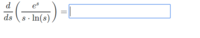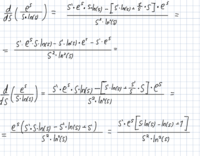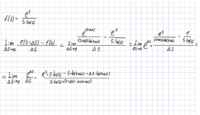# Derivative calculating help

#### akleron

##### New member
hello !
I'm trying to calculate the derivative in the following question.
I've tried both with derivative rules and derivative definition but didn't get too far.
1st picture is the question
2nd is what I've tried to do.
Would love some help, thanks !#### Romsek

##### Full Member
well let's break it down

$$\displaystyle \dfrac{d}{ds}e^s = e^s$$

$$\displaystyle \dfrac{d}{ds} s \ln(s) = \ln(s) + 1$$

$$\displaystyle \dfrac{d}{ds}\dfrac{e^s}{s\ln(s)}= \dfrac{e^s(s \ln(s)) - e^s(\ln(s)+1)}{(s\ln(s))^2} = \\~\\ \dfrac{e^s}{s \ln(s)} - \dfrac{e^s}{s^2\ln(s)} - \dfrac{e^s}{s^2(\ln(s))^2}$$

you seemed to miss the fact that $$\displaystyle \dfrac{d}{ds} s = 1$$

•Jomo, Subhotosh Khan and akleron

#### akleron

##### New member
well let's break it down

$$\displaystyle \dfrac{d}{ds}e^s = e^s$$

$$\displaystyle \dfrac{d}{ds} s \ln(s) = \ln(s) + 1$$

$$\displaystyle \dfrac{d}{ds}\dfrac{e^s}{s\ln(s)}= \dfrac{e^s(s \ln(s)) - e^s(\ln(s)+1)}{(s\ln(s))^2} = \\~\\ \dfrac{e^s}{s \ln(s)} - \dfrac{e^s}{s^2\ln(s)} - \dfrac{e^s}{s^2(\ln(s))^2}$$

you seemed to miss the fact that $$\displaystyle \dfrac{d}{ds} s = 1$$
Lol should've know that (s)'=1 ...
Thank you !

#### Harry_the_cat

##### Senior Member
Also, should be -1 not +1 in square bracket.

#### HallsofIvy

##### Elite Member
What were all those "s' "s in your answer? Was that supposed to be the derivative of s? Since you were differentiating with respect to s, s'= 1.